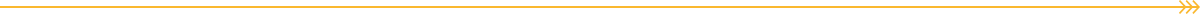# Katie OliverasKatie Oliveras set out to create a course that made graph theory accessible through studying the complexity of pandemics. The course makes use of animated gifs to illustrate complicated mathematical concepts. As a UCOR class, Katie wanted to ensure students were given plenty of feedback through interactive quizzes focused on gaining mastery of new concepts. Each module takes students through a set of concepts illustrated with videos and animated gifs created by Katie. Her illustrative and informal style is at once accessible and clear and works together explain complex concepts to novice learners. Because the content builds upon itself, Katie wanted to make sure students had ample opportunity for feedback, which she built into Canvas quizzes. Below is a sample module set up that students could click through, an example animated GIF, and one of the quiz questions she asks students to answer.EXAMPLE CONTENTExample. The animated gif shown below shows a circuit of length 4 that begins and ends at vertex A.If we wanted to write out the path, we would simply list the vertices in order: A, B, C, F, A.

Note that in this example, we listed the vertex A at the beginning and at the end.  Since A is at the beginning and end of the list, we know that the list represents a circuit and not a path.

 Starting Vertex Circuit Mirror Image A A, B, C, F, A A, F, C, B, A B B, C, F, A, B B, A, F, C, B C C, F, A, B, C C, B, A, F, C F F, A, B, C, F F, C, B, A, F